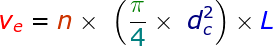# Engine Swept Volume Calculator

Engine swept volume or swept volume in general can be explained as the volume required to complete the stroke movement of the piston from TDC to BDC. We can find engine swept volume by multiplying the swept volume times number of pistons in an engine.

Here is the formula:where,
ve = Engine swept volume [cm3]
n = number of cylinders
dc = cylinder diameter [cm]
L = stroke length [cm]

Enter the required fields in the below calculator and press calculate button to find the output.

 Cylinder Diameter: [cm] Stroke Length: [cm] Number of Cylinders: Cylinder Swept Volume: [cm3]

Latest Calculator Release

Average Acceleration Calculator

Average acceleration is the object's change in speed for a specific given time period. ...

Free Fall Calculator

When an object falls into the ground due to planet's own gravitational force is known a...

Torque Calculator

Torque is nothing but a rotational force. In other words, the amount of force applied t...

Average Force Calculator

Average force can be explained as the amount of force exerted by the body moving at giv...

Angular Displacement Calculator

Angular displacement is the angle at which an object moves on a circular path. It is de...